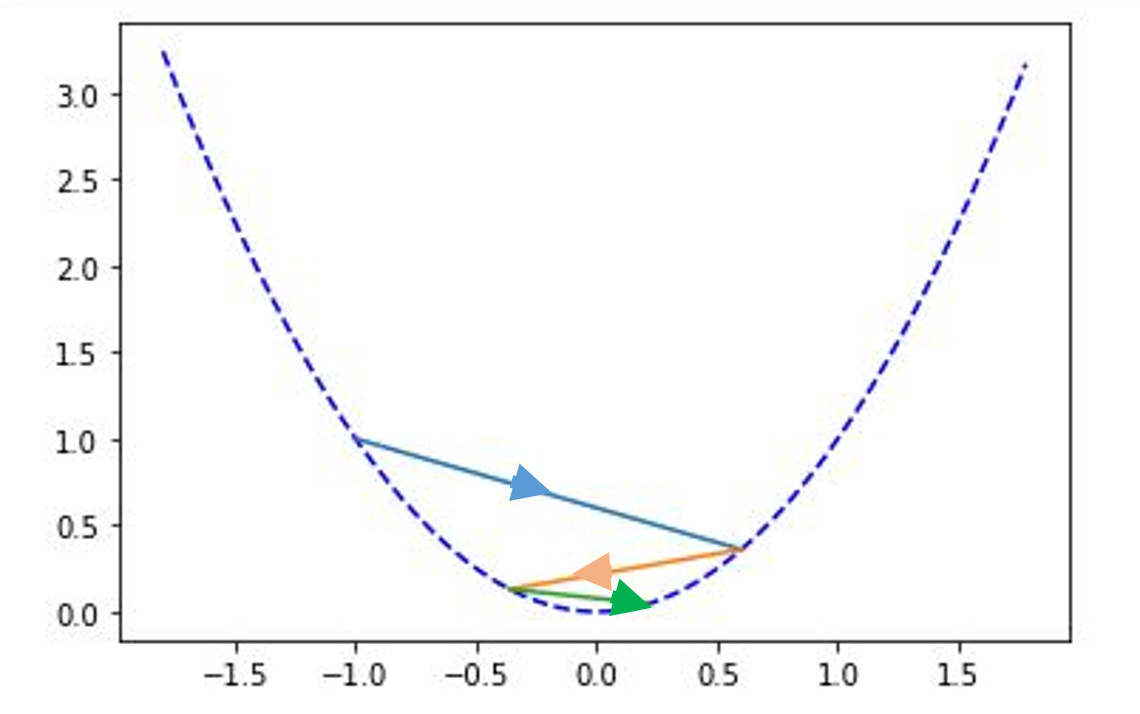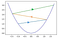# Why do high learning rate diverges the weight updates?

This has been one of the intriguing questions that I faced while learning about the learning rates. I always used to feel that even if learning rate was large, the model should just oscillate about the optimal point. There didn’t seem any reason for it to start diverging, until I decided to work the maths up. And it turns out that it is pretty easy, just have to use a bit of optimization techniques from mathematics.

So, as an example, let’s assume that the function that we want to minimize is y = x². We know that the minima occurs at x = 0 for this function. Let’s say we are at x = -1 currently, and we have to reach x = 0 for minimizing our cost function. Now, just for the sake of completeness in this post, let’s recall the gradient descent step to update the value of x:

x’ = x — learning_rate * partial derivative of cost function wrt x

As we can easily see, our cost function here is x² and the derivative of x² wrt x is 2x. So, the gradient step becomes:

x’ = x (1–2*learning_rate)

Ok, so we have our update formula now and remember that we are at x = -1 currently. So, to see why weights diverge, we’ll use two values of learning rate. Let’s start with learning rate = 0.8 and we will do 3 update steps.

So, after first step x’ = (-1) * (1–2*0.8) = 0.6.
After second step, x’ = 0.6 * (1–2*0.8) = -0.36
After third step, x’ = -0.36 * (1–2*0.8) = 0.216
The graph of this looks something as below:Gradient descent from x = -1 for learning rate of 0.8

Ok, so far so good.That’s what we expected. So,now let’s see with a learning rate of 1.2.
So, after first step x’ = (-1) * (1–2*1.2) = 1.2.
After second step, x’ = 1.2* (1–2*1.2) = -1.44
After third step, x’ = -1.44 * (1–2*1.2) = 1.728
The graph of this looks something as below:Gradient descent from x = -1 for learning rate of 1.2

Wait what’s that. The graph starts to diverge. Why is it so.

Well, the main reason is that the learning rate becomes so high, that when we take one step, though we move from one side of minima to another, but we increase the absolute value of x. And since the graph is symmetrical about x = 0, the value of y= x² increases and our cost function starts to diverge.

The same is true for a machine learning model as well, just that the loss function won’t be this straight forward. Just for the kicks, one can try to see what happens if we took the learning rate to be 1.

So, now we understand why it is extremely important to tune the learning rate for our model.

I will be extremely happy to hear your views about this or any other feedback, since this is my first attempt at blogging.

So long!

## More from Prash goel

Software Engineer. Deep Learning. Autonomous cars.